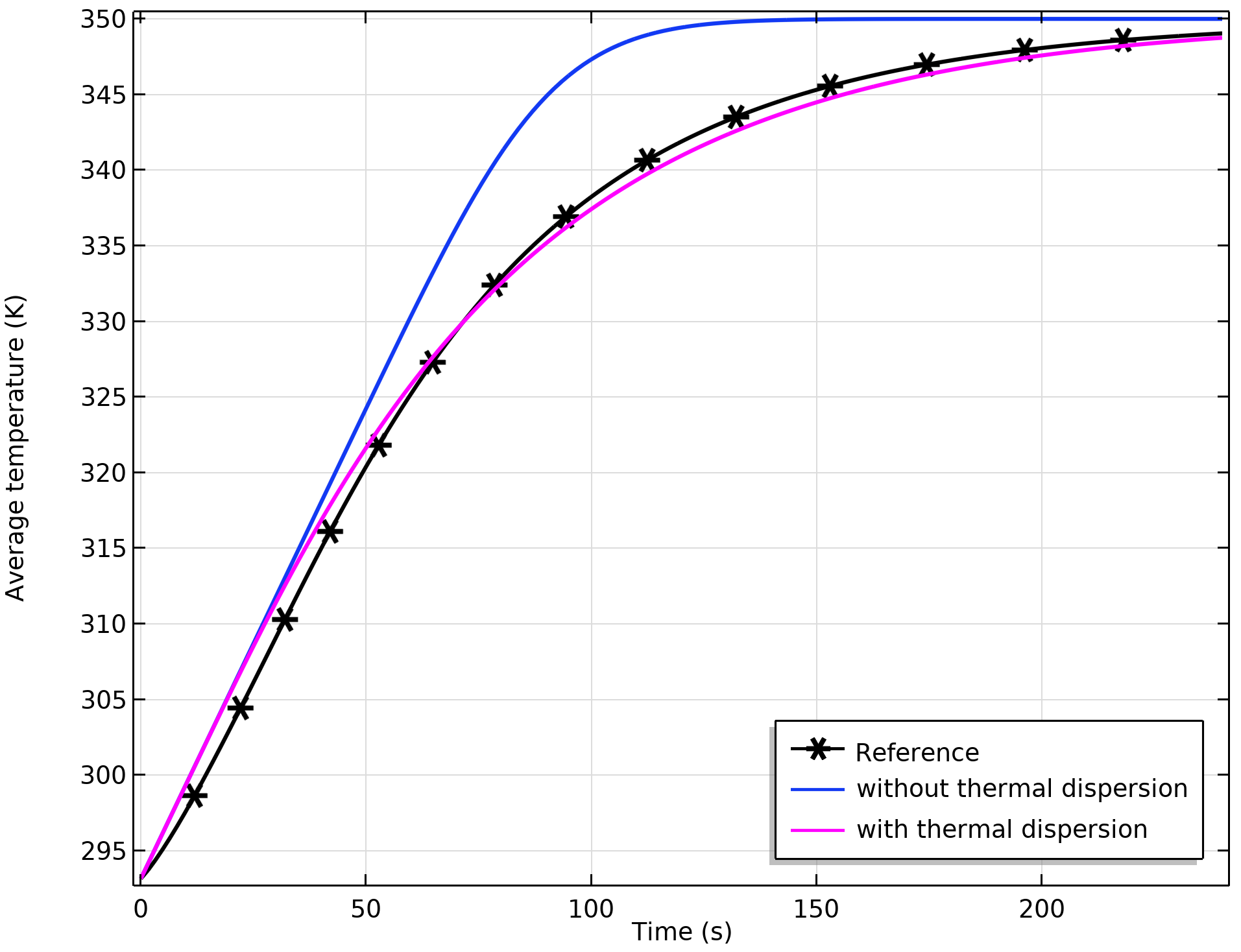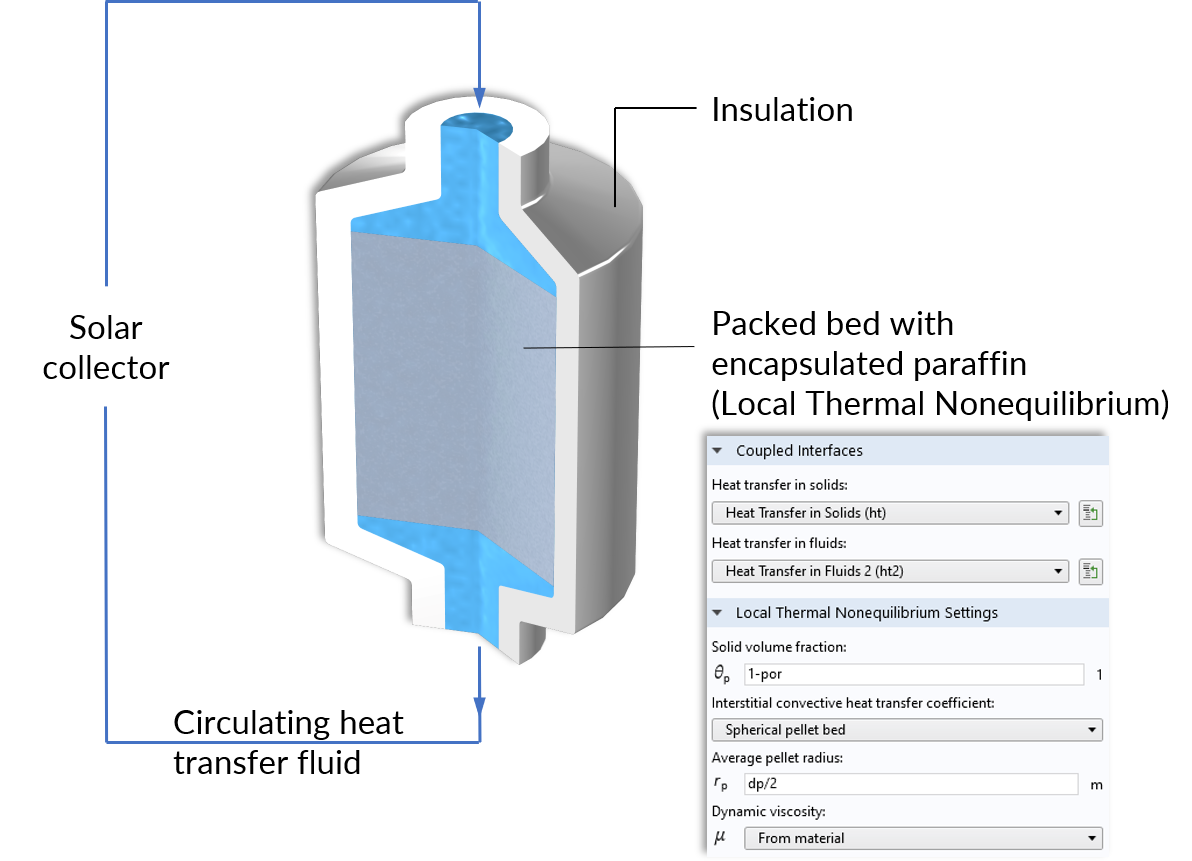# 多孔介质中的热平衡与热非平衡传热

2020年 3月 3日

### 热平衡下的热传递

(1)

\left(\rho C_p\right)_\textrm{eff}\frac{\partial T}{\partial t}+\rho_\textrm{f} C_{p,\textrm{f}} \mathbf{u}\cdot\nabla T+\nabla\cdot(-k_\textrm{eff}\nabla T)=Q

\left(\rho C_p\right)_\textrm{eff}=\theta_\textrm{p}\rho_\textrm{s}C_{p,\textrm{s}}+\theta_\textrm{f}\rho_\textrm{f}C_{p,\textrm{f}}

1. 体积平均值，代表与热通量平行的固体和流体条纹 k_\textrm{eff}=\theta_\textrm{s} k_\textrm{s} + \theta_\textrm{f} k_\textrm{f}
2. 倒数平均值，垂直于热通量的固体和流体条纹 \frac{1}{k_\textrm{eff}}=\frac{\theta_\textrm{s}}{k_\textrm{s}} + \frac{ \theta_\textrm{f}}{k_\textrm{f}}
3. 幂定律，对于固体和流体具有相似热导率的随机几何k_\textrm{eff}=k_\textrm{s}^{\theta_\textrm{p} }\cdot k_\textrm{f}^{\theta_\textrm{f}}

### 热分散### 热非平衡下的热传递

\begin{align*}
\theta_\textrm{s}\rho_\textrm{s} C_{p,\textrm{s}} \frac{\partial T_\textrm{s}}{\partial t} + \nabla\cdot(-\theta_\textrm{s} k_\textrm{s} \nabla T_\textrm{s}) &= q_\textrm{sf}(T_\textrm{f}-T_\textrm{s})\\
\theta_\textrm{f}\rho_\textrm{f} C_{p,\textrm{f}} \frac{\partial T_\textrm{f}}{\partial t}+\rho_f C_{p, \textrm{f}}\mathbf{u}\cdot\nabla T_\textrm{f} + \nabla\cdot(-\theta_\textrm{f} k_\textrm{f} \nabla T_\textrm{f})&=q_\textrm{sf}(T_\textrm{s}-T_\textrm{f})
\end{align}### 参考文献

1. D. A. Nield and A. Bejan, Convection in Porous Media, 4th ed. Springer, 2013.